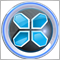# Array out of range in zigzag3136

hi guys, im trying to catch zigzag values for each symbol, but i get the "Array out of range" error on the loop that is using for catching zigzag values.

here is the function for catching zigzag:

```//+--------------------------------------------------------------
void zig(string symbol, int Depth,int Deviation,int Backstep, int shift1)
{
double z;
int ct=0;

for(i=shift1 ; i<shift1+500 ; i++)
{
z=iCustom(symbol,ZigTF,"ZigZag",Depth,Deviation,Backstep,0,i); // ZigTF is a selectable input ENUM_TIMEFRAME
if(z!=0 && ct==1)
{
value2=z;
break;
}
if(z!=0 && ct==0)
{
value1=z;
ct++;
}
}

}
//+------------------------------------------------------------------+```

and here is the loop that i use for changing symbols (witch is not the problem because i Resize the symbol array before):

```     for(i=0;i<ArraySize(aSymbols);i++)
{
zig(aSymbols[i],InpDepth,InpDeviation,InpBackstep,0);

}```

can you help me to know whats the problem?28718

So in the loop of Zig function there is not any array that to be cause of the error,

Which line is the error marking?the code is incomplete,it can't be easy to figure it out.148114

hi guys, im trying to catch zigzag values for each symbol, but i get the "Array out of range" error on the loop that is using for catching zigzag values.

here is the function for catching zigzag:

and here is the loop that i use for changing symbols (witch is not the problem because i Resize the symbol array before):

can you help me to know whats the problem?

` for(i=shift1 ; i<shift1+500 ; i++)`3136

So in the loop of Zig function there is not any array that to be cause of the error,

Which line is the error marking?the code is incomplete,it can't be easy to figure it out.

your right mehrdad, i checked the entire code again and noticed the "i" value that handles my pairs loop, is changing in the end of code by another function, and its new value is greater than my pair array size. so i rename it to "j" and the problem solved.
thanks guy.28718